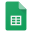# Lime render or plaster calculator

## The calculator

### Motivation

I developed the spreadsheet below as a consolidation of what I've learned and a response to some fundamental errors I'd found on other web sites, some of which I've detailed. There are also some details of the calculations used.

### Get started

Open the spreadsheet in a separate tab or window to see Row and column headings and the menu bar.

Navigate to Files->Download and choose the file type to download as: ODS if you have OpenOffice or LibreOffice, or XLSX if you have Excel. You can't use the spreadsheet without downloading it. I know that ODS works, including the Quantities graph that is not critical if it doesn't, but you may have to choose Edit Spreadsheet there before you can enter or change values. I haven't tried Excel.

Just enter a coverage area and coat depth, measure the void space in your sand/aggregate and enter the mix ratio you want to use, which may also depend on the void space measurements.

To measure void space I use the saturation method where water is added to a known volume of sand until it saturates with no excess water. You should also check the after stirring and knocking/vibrating the sand down during this process to duplicate the effect of mixing because it will change the result slightly. And of course, these measurements must be done just prior to mixing. A day can make a difference.

### Moisture content % (by weight)

First of all, measure the moisture content of the sand and enter that result into the Moisture % cell in the Porosity measurement table. How to:

1. Take a sand sample (the larger the better for accuracy) and weigh it. This is "S(weight1)".
2. Oven-dry the sample then weigh it again. This is "S(weight2)".
3. Calculate the moisture content % as: 100*(S(weight1) - S(weight2))/S(weight2)

If you want to guess, moisture can range typically from 2% to 6%.

### Porosity ratios

I will describe a method that I think will achieve the two measurements with a single sample:

1. fill the measuring cylinder with loose sand and read off the level as SAND(loose). We know that the volume and weight of the actual solids will remain constant.
2. measure W(initial) equivalent to SAND(loose) of water and pour it in, shake, stir and tap as it settles, to compact the solids.
3. read off the volume at the top of the water and top of the solids. These are SAND(compact) and W(cover).

4. Then:

WATER(compact) = SAND(compact) + W(initial) - W(cover)

Because the actual solids (Silicon) volume and weight remain constant:

WATER(loose) = SAND(loose) + WATER(compact) - S(compact)

5. These values can now go into the spreadsheet in the Porosity measurement table:

• "Loose" SAND = SAND(loose)
• "Loose" WATER = WATER(loose)
• "Compacted" SAND = SAND(compact)
• "Compacted" WATER = WATER(compact)

An example:

SAND(loose) = 200

W(initial) = 200

W(cover) = 320

SAND(compact) = 175

WATER(compact) = SAND(compact) + W(initial) - W(cover)

= 175 + 200 - 320

= 55

WATER(loose) = SAND(loose) + WATER(compact) - SAND(compact)

= 200 + 55 - 175

= 80

### Goal seeking calculations

If you don't want to start with coverage but instead want to calculate, say, an exact tonne of render, or how much sand to go with 25kg of lime putty, then you can use the goal seek tool in OpenOffice, LibreOffice or Excel. In the goal seek panel just set the cell that shows your known quantity as the target cell, enter the known value as the target value in the goal seek panel and choose the coverage area cell as the variable value. You can goal seek any value.

The calculation assumes lime putty will be used. If you want to use dry hydrated lime then I suggest turning it into putty and maturing it before mixing it to a render. If you use hot lime mixes then I assume you don't need this spreadsheet.

### Mix ratios

Please take note: the ratios in the Mix table are relative to "compacted" sand. So that the correct equivalent amount of loose sand is put into the mixer, the Quantities table shows a different ratio, which is relative to loose sand. However, the weights of the sand and lime in both conditions remains the same.

If you have measured the porosity and moisture content as above the spreadsheet will also calculate a "perfect" mix ratio, where the lime exactly equals the compacted sand voids. You can choose to use that ratio or use your custom ratio to compute the quantities of sand and lime putty required. Select which mix ratio you want to use by leaving the highlighted cell to the right of the "Perfect ratio" blank or not.Lime render calculation